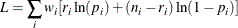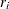Computational Method

The log-likelihood function is maximized by means of a ridge-stabilized Newton-Raphson algorithm. Initial regression parameter estimates are set to zero. The INITIAL= and INTERCEPT= options in the MODEL statement can be used to give nonzero initial estimates.

The log-likelihood function, L, is computed aswhere the sum is over the observations in the data set,is the weight for the ith observation, andis the modeled probability of the observed response. In the case of the events/trials syntax in the MODEL statement, each observation contributes two terms corresponding to the probability of the event and the probability of its complement:whereis the number of events andis the number of trials for observation i. This log-likelihood function differs from the log-likelihood function for a binomial or multinomial distribution by additive terms consisting of the log of binomial or multinomial coefficients. These terms are parameter-independent and do not affect the model estimation or the standard errors and tests.

The estimated covariance matrix,, of the parameter estimates is computed as the negative inverse of the information matrix of second derivatives of L with respect to the parameters evaluated at the final parameter estimates. Thus, the estimated covariance matrix is derived from the observed information matrix rather than the expected information matrix (these are generally not the same). The standard error estimates for the parameter estimates are taken as the square roots of the corresponding diagonal elements of.

If convergence of the maximum likelihood estimates is attained, a Type III chi-square test statistic is computed for each effect, testing whether there is any contribution from any of the levels of the effect. This statistic is computed as a quadratic form in the appropriate parameter estimates by using the corresponding submatrix of the asymptotic covariance matrix estimate. See Chapter 42: The GLM Procedure, and Chapter 15: The Four Types of Estimable Functions, for more information about Type III estimable functions.

The asymptotic covariance matrix is computed as the inverse of the observed information matrix. Note that if the NOINT option is specified and classification variables are used, the first classification variable contains a contribution from an intercept term. The results are displayed in an ODS table named Type3Analysis.

Chi-square tests for individual parameters are Wald tests based on the observed information matrix and the parameter estimates. If an effect has a single degree of freedom in the parameter estimates table, the chi-square test for this parameter is equivalent to the Type III test for this effect.

Prior to SAS 8.2, a multiple-degrees-of-freedom statistic was computed for each effect to test for contribution from any level of the effect. In general, the Type III test statistic in a main-effect-only model (no interaction terms) will be equal to the previously computed effect statistic, unless there are collinearities among the effects. If there are collinearities, the Type III statistic will adjust for them, and the value of the Type III statistic and the number of degrees of freedom might not be equal to those of the previous effect statistic.

The theory behind these tests assumes large samples. If the samples are not large, it might be better to base the tests on log-likelihood ratios. These changes in log likelihood can be obtained by fitting the model twice, once with all the parameters of interest and once leaving out the parameters to be tested. See Cox and Oakes (1984) for a discussion of the merits of some possible test methods.

If some of the independent variables are perfectly correlated with the response pattern, then the theoretical parameter estimates can be infinite. Although fitted probabilities of 0 and 1 are not especially pathological, infinite parameter estimates are required to yield these probabilities. Due to the finite precision of computer arithmetic, the actual parameter estimates are not infinite. Indeed, since the tails of the distributions allowed in the PROBIT procedure become small rapidly, an argument to the cumulative distribution function of around 20 becomes effectively infinite. In the case of such parameter estimates, the standard error estimates and the corresponding chi-square tests are not trustworthy.